# Income tax calculating formula in Excel

First of all, you need to know that – in some regions, income tax is taken by your company accountant from your income. Conversely, you need to calculate the income tax by yourself in some regions. Thus, this tutorial will help both users to calculate income tax in Excel.

You can keep all details of your income, expenses, and expenditure in an Excel worksheet. From here, you can easily calculate the income tax on the stored data. We will show you two ways to calculate income tax.

1. Calculate taxable income and income tax
2. Calculate taxable income and income tax using income slabs

Excel provides other solutions using Excel in-built functions to calculate income tax, which is –

• Calculate the income tax using IF or nested IF
• Calculate the income tax using the VLOOKUP function

We will describe each method one-by-one.

## What is Income tax?

Income tax is a tax taken by the central government on your income earned during the financial year. This financial year starts from April 1 and ends on March 31 every year.

Income tax is a direct tax imposed on an individual or business. Individual’s income or business’s profit. It is the official website of https://incometaxindia.gov.in/ income tax. In Excel, it requires a lengthy formula to calculate income tax. It is a bit tricky and lengthy calculation.

## Gross income and taxable income

Usually, gross income and taxable income both are different. However, it can be same sometimes. For this, understand what is gross income and taxable income.

Gross income is the income coming from all the income sources. For example, salary, house rent, FDs, and all. While taxable income is extracted from the gross income by excluding exemptions and deductions.

## Prerequisites before calculating Income tax

Before you start calculating the income tax on your income, the users must know all the important terms related to income tax. So, he/she can calculate it correctly.

1. To calculate the income tax, the user must know the latest income tax slab.
2. In addition, the user should also know the tax rates to be put on income.
3. Last date to pay income tax.
4. You can pay the tax online by yourself from the official income tax website or pay it to the income tax office.
5. Gross income comes from all the income sources.
6. There are no exemptions and deductions, then the gross income will be the Taxable income.
7. One should also be aware with the educational tax.

## Calculate the income tax and taxable income

Firstly, we will show you to extract the taxable income from the gross income and then calculate the income tax on taxable income. See the steps to get the taxable income and income tax. Do not forget to define the tax percentage to apply on income.

It is a simple way to calculate income tax and easy to understand.

Step 1: Open the Excel worksheet that contains the income details of someone. We have this income dataset: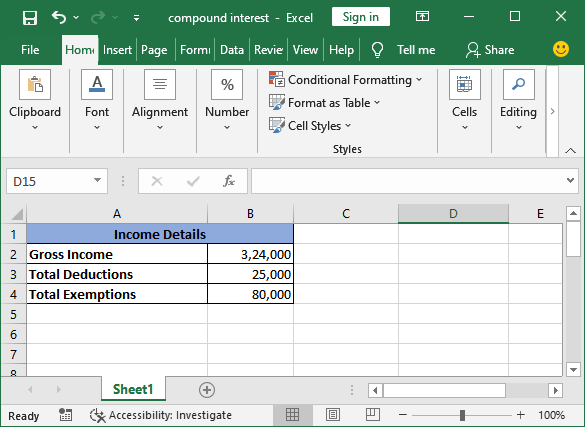Step 2: As we want to calculate the taxable income and tax. So, create two rows: one for the taxable value and another for the tax.Step 3: Select a cell where you want to place the calculated result and subtract the expenses (exemptions and deduction) from the gross income.

Write the formula =B2-B3-B4 inside the formula bar and press the Enter key.Step 4: Taxable income is now extracted from gross income, which is 2,19,000.

“Taxable income is on which we apply the tax.”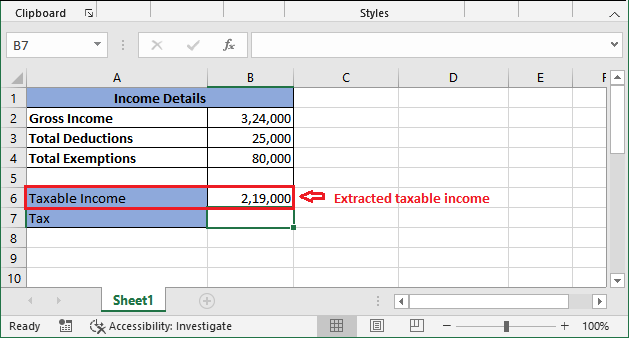“Tax is 5% on income below 2,50,000.” As the taxable value is between 1.5 to 2.5 lakhs so that 5% will apply to income.

Step 5: Now, calculate the tax on extracted taxable value. Apply the following formula in cell B7: =B6*5/100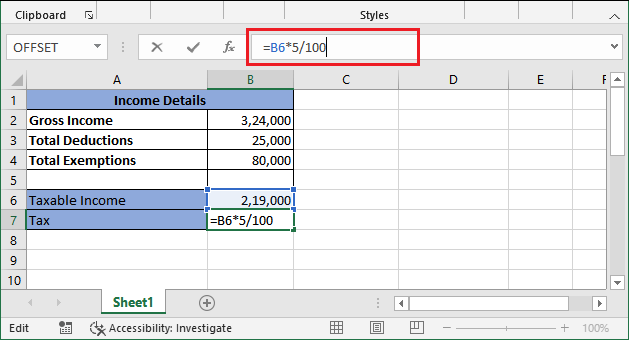Step 6: Press the Enter key and get the income tax value calculated on the taxable income. See that the returned tax value is 10,950.Conclusion: Now, the summary for the above data is – for this income, this user needs to pay 10950 rupees as the income tax.

## Calculate the income tax with income slabs

Now, we will show another example to calculate the income tax with different income taxes. We have income slabs to be applied on income data.

These income slabs are defined by the government for different ranges of income. According to these slabs, tax percentage varies and calculated. Let’s see an example and detailed steps for this type of tax calculation.

Step 1: We have this dataset of someone’s income and income slabs provided by the government.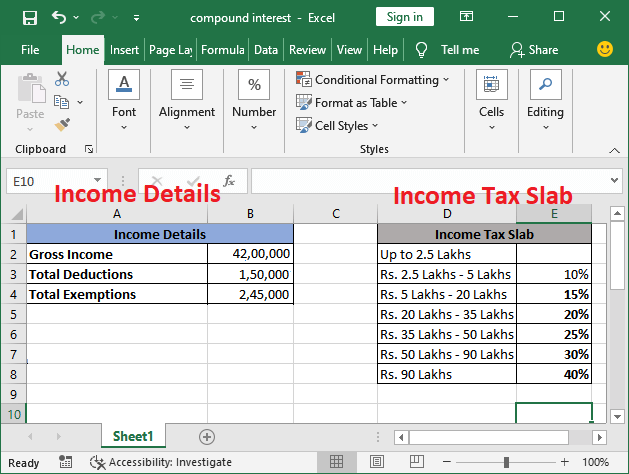Now, we will calculate the income tax according to these slabs. Similar to the above method, we will calculate taxable income and tax.

#### Note: Till 2.5 Lakhs of income, tax is NIL.

Step 2: For this, we will define two rows: one for the taxable value and another for the tax.Step 3: Firstly, we need to extract the taxable value on which the tax will calculate. So, we will subtract the exemptions and deduction from the gross amount.

For this, write the formula =B2-B3-B4 inside the formula bar and press the Enter key.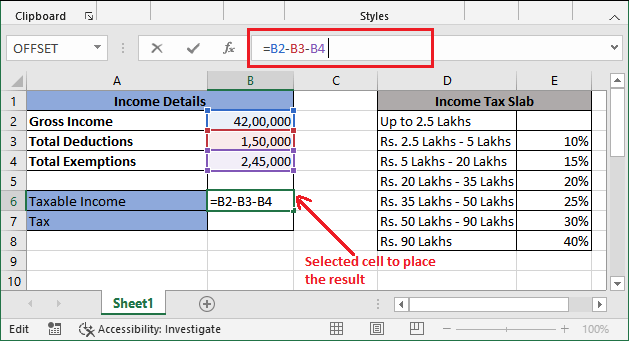Do not forget to select the respective cell to place the calculated result.

Step 4: Taxable income is now extracted from gross income, which is 38,05,000.

“Taxable income is on which we apply the tax.”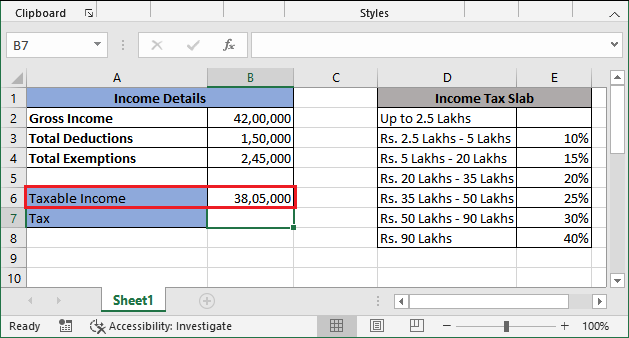“This time different Tax slabs are defined (see at right side in Excel sheet) for calculating the tax.” Since the taxable value is laying between 35 lakhs to 50 Lakhs (5th slab). So, the tax will be calculated till the 5th slab, i.e., 10%, 15%, 20%, and then 25% on income.

Income slab Calculation

This will definitely the easiest way to calculate income tax through income tax.

Step 5: Find out the total tax to be calculated for each slab up to the targeted one. Remember – Tax is NILL till the income of 2.5 lakhs. Hence, the tax will be computed for the income above 2.5 Lakhs.Step 6: Now, we will calculate the tax for each defined slab here using the formula.

=taxable income*tax_rate/100

Step 7: See the calculation for the first slab. The other three will be calculated the same by changing the taxable value and tax rate in the formula.Step 8: Press the Enter button and get the result of 10% tax over 2.5 Lakhs. See it below: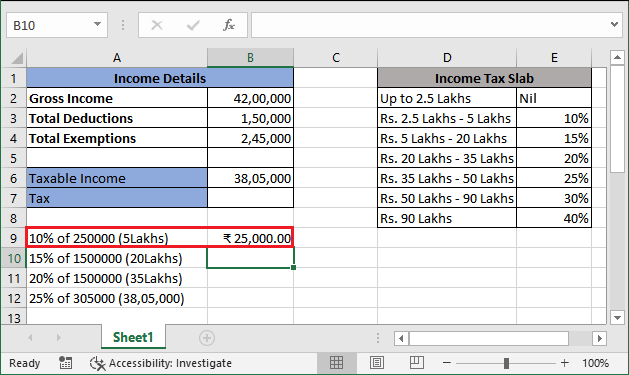Step 9: Similarly, calculate the tax for all other defined rows by changing the taxable value and tax rate in the formula. See the screenshot below:Step 10: The final step is – add the extracted tax through the different slabs and get the tax value, which needs to be paid by the taxpayer. For our data –

=B9+B10+B11+B12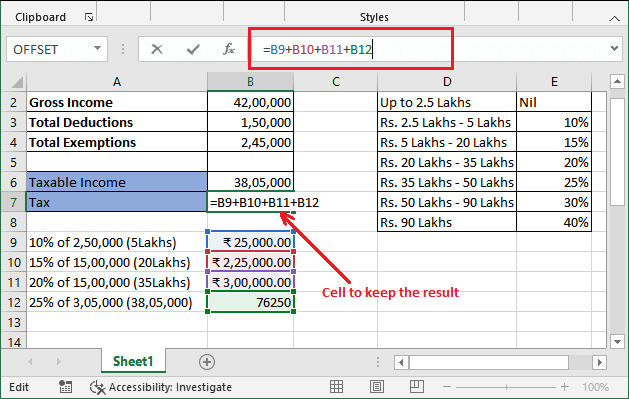Step 8: Press the Enter button and get the final tax value for the taxable income with income slabs, which is 6,26,250 rupees total.Income slab Calculation interpretation

In some tutorials, you will see that they have applied the formula only according to slab directly to calculate income tax: taxable income*25/100 as value lies in 5th income slab.

For example –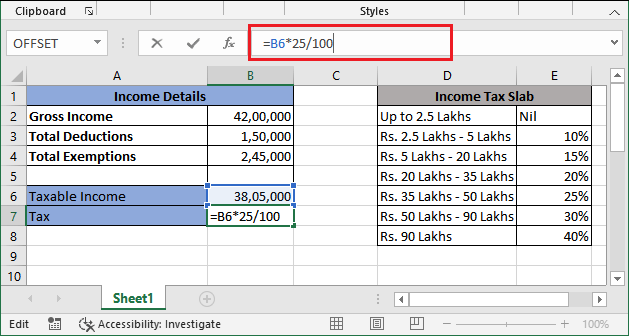Step 6: Press the Enter key and get the income tax value calculated on the taxable income. See that the returned tax value is 9,51,125.Additionally, this calculation goes wrong for each income there is a different tax rate defined. Thus, the users have to calculate the tax for each slab individually until the slab where the taxable value lies.

Conclusion: In the end, the summary for the given data (taxable income and tax slab) is – for this income, this user needs to pay 6,26,250 rupees, not 9,51,250 rupees as the income tax.

## Calculate the income tax using IF or nested IF

IF function also helps the Excel users to calculate the tax in a single calculation like the above one. The formula of income tax becomes lengthy but provides the result fast. Let us see an example of calculating income tax using the IF function.

Syntax for IF function

We have the following set of data of some people’s income. We will calculate the tax on their income using nested IF (IF inside IF).We have prepared this Excel worksheet to calculate income tax on different ranges of income. These all given incomes are taxable income.

Calculate tax on Martina’s Salary: 380000

Step 1: To calculate the tax on Martina’s income, select the C10 cell to keep the result and write the following IF formula in the formula bar.

=IF(B10

To see the explanation how this formula is created, click here. (Explanation of income tax formula using nested IF)Step 2: Now, hit the Enter button and see the returned value for the calculated tax on Martina’s salary amount. See that Tax is NiL.Now, we will calculate the tax on Harry’s salary, whose salary amount is 17,00,000. You do not need to create the whole formula again. By modifying one change in the previous formula, you can calculate the tax for other’s income.

#### Note: Tax slab should be same.

Calculate tax on Harry’s Salary: 17 Lakhs

Step 1: To calculate the tax on Martina’s income, select the C11 cell to keep the result and write the same IF formula as the above one by just replacing the B10 cell number to B11.

=IF(B11Step 2: Hit the Enter button and get the tax calculated for Harry’s salary. See that the returned tax value for the Harry’s salary is 1,80,000 rupees on 17Lakhs of income.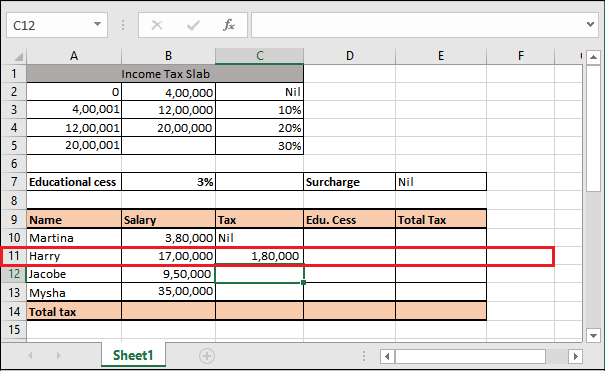Now, the same steps will follow for the Jacobe and Mysha tax calculation. Make the changes in formula and get the calculated result. However, we will provide the formula as well.

Calculate tax on Jacobe’s Salary: 9,50000

Calculate tax on Mysha’s Salary: 35,00,000

See the final worksheet after calculating the tax for all given employee’s data.### Apply Educational Tax (cess)

Tax on all incomes have been calculated successfully. Now, the next step is to apply education tax (cess), i.e., 3%. This tax will compute on the income tax (collected from income). If the income tax is NIL on someone’s income, educational cess will also be 0 by default. However, you can also calculate it using the formula.

This time, it does not require a lengthy calculation. It needs only two conditions to be checked inside the IF function. Follow the steps below:

Step 1: Select the D10 cell to keep the returned result and write the following formula to compute the educational tax firstly on Martina’s income tax value (C10 cell).

=IF(C10=”Nil”, 0, C10*3%)Step 2: Hit the Enter button and see that the educational tax is 0 for Martina’s income tax.We will show you for one record, i.e., Harry’s income tax.

Step 3: Select the D11 cell to keep Harry’s educational tax result and write the following formula to compute the educational tax on Harry’s income tax (C11 cell).

=IF(C11=”Nil”, 0, C11*3%)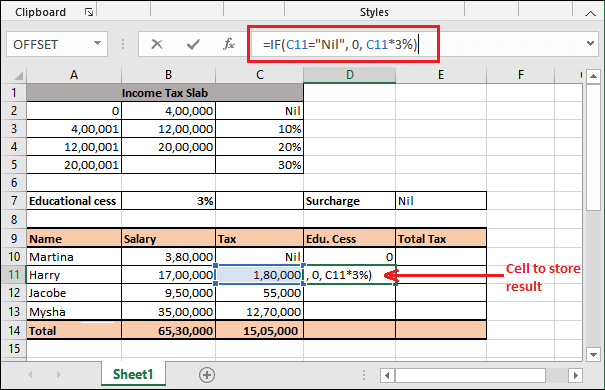Step 4: Hit the Enter key and get the educational cess for Harry’s tax value. See that – it has returned 5,400.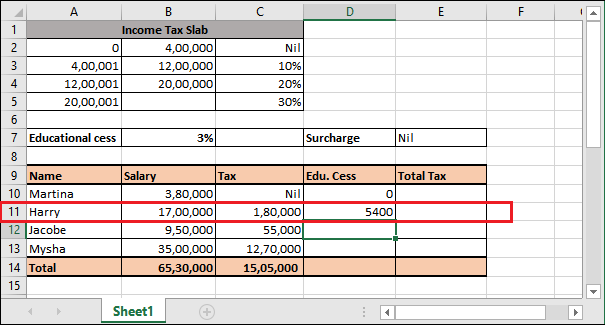Educational tax on Jacobe’s income tax: 1,80,000

Educational tax on Mysha’s income tax: 12,70,000

Step 5: See that worksheet after finding educational tax on all data.Step 6: Add the income tax and educational tax and store the result in E column.

Note that the total tax is 15,50,150 on total income of Martina, Harry, Jacobe, and Mysha 65,30,000.So, these are the complete steps to calculate the income tax on someone’s income. Similarly, you can compute for another dataset.

### Explanation of income tax formula using nested IF

We have created a formula to calculate the income tax using the IF function. Although it is lengthy, but you can use the same data for all data given in this worksheet but by making a small change.

See the explanation for it. This will help you to understand how this formula is created.

B11 It will check that if the condition B11true, the tax will be NiL; otherwise next given condition will check. B11

B11<=B3: It defines that if this condition comes true, first slab amount will be subtracted from the salary amount and then multiply with the defined tax rate percentage, i.e., 10%. If the condition finds false, we will move to the next nested IF condition check.

B11<=B4: It refers to that if this condition (B11<=B4) comes true, the second slab amount will be subtracted from the salary amount, then multiple with the defined tax rate percentage, i.e., 20%. Additionally, 10% on the previous taxable amount will also add to this.

If the condition finds false, we will move to the else part as it is the last slab. Now, the third slab amount will be extracted from the given salary amount and the final tax rate (30%) tax will apply to the remaining amount. Besides this, 10%+20% (i.e., 2,80,000) on previous taxable accounts will also add to this.

## Calculate the income tax using VLOOKUP function

The above calculation can also take place using the VLOOKUP function of Excel. Income tax can be calculated using the VLOOKUP function of Excel, but it is a bit lengthy and confusing.

Income tax formula using VLOOKUP is not a simple formula; you need to follow a bit lengthy calculation. You can use VLOOKUP to calculate income tax on a certain income in Excel.

### Syntax for VLOOKUP

To understand the VLOOKUP formula, you should know the syntax of the VLOOK() function.

### Steps to calculate income tax using VLOOKUP

Step 1: We use the same dataset as used in the nested IF example and the calculated income tax is also still there available.

We will just calculate the income tax for Jacobe salary to verify and show that the tax value return by the VLOOKUP() function will be the same as the IF() function.Step 2: Now, select a cell to keep the result and write the following LOOKUP formula inside the formula bar.

=VLOOKUP(B12,A2:C5,3,TRUE)+(B12-VLOOKUP(B12,A2:C5,1,TRUE))*VLOOKUP(B12,A2:C5,3,TRUE)Step 3: Hit the Enter key and see that the return tax value through the VLOOKUP formula is the same as the tax calculated using a nested-IF function, i.e., 55,000.Hence, the VLOOKUP formula works like that. Hope you get the different methods to calculate the income tax in Excel.

,”nil”,>

,”nil”,>